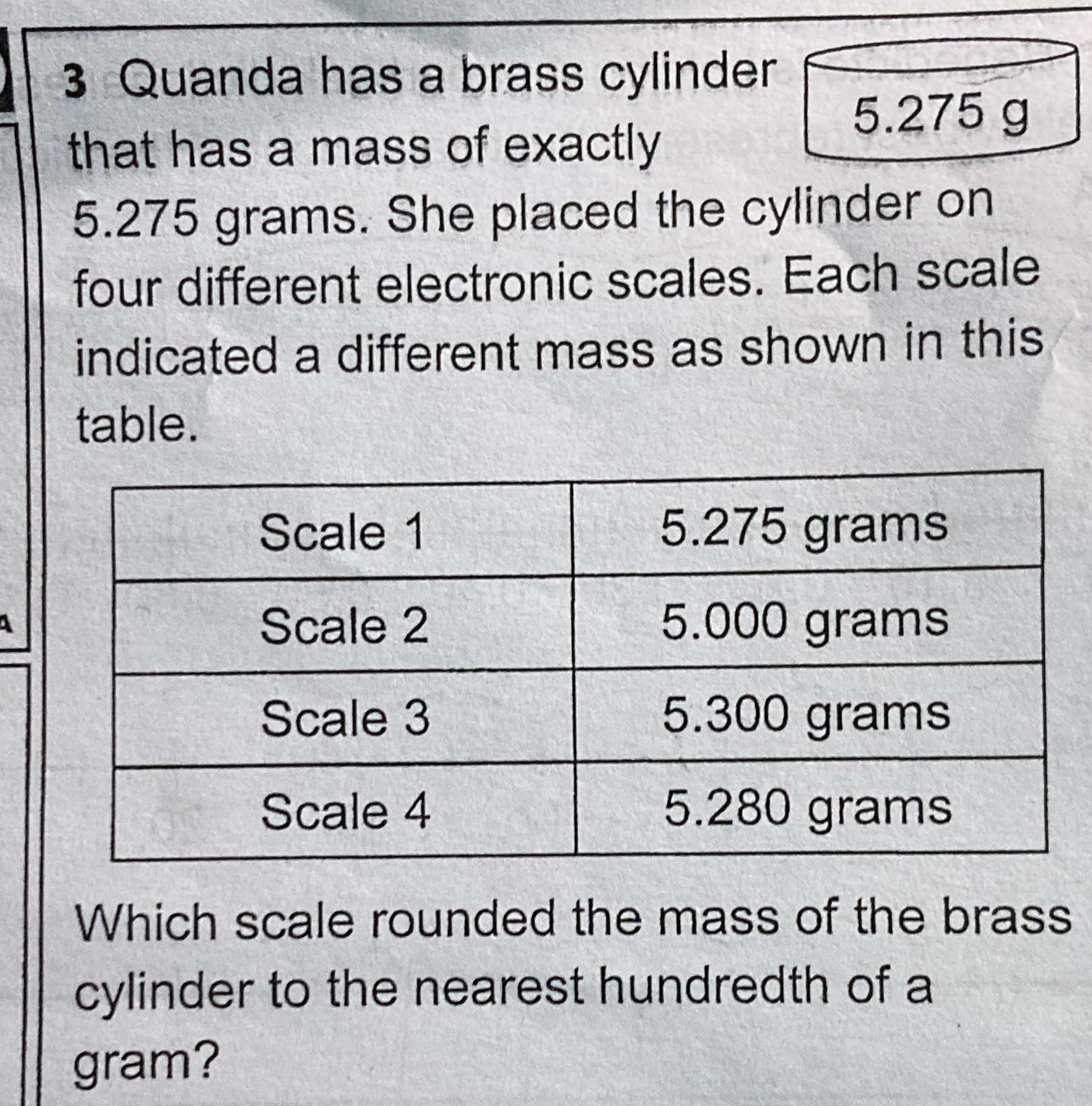### Still have math questions?

Arithmetic
Question$$3$$ Quanda has a brass cylinder that has a mass of exactly $$5.275$$ grams. She placed the cylinder on four different electronic scales. Each scale indicated a different mass as shown in this table.

Scale1    5.275grams

Scale2    5.000grams

Scale3    5.300grams

Scale4    5.280grams

Which scale rounded the mass of the brass cylinder to the nearest hundredth of a gram?